# berryFunctions

#### 2022-05-13

The R package berryFunctions, available at github.com/brry and CRAN, contains my collection of miscellaneous functions. A lot is related to plotting and hydrology (Vignette Rmd source).

## Package installation

install.packages("berryFunctions")
library(berryFunctions)

To get the development version on github, including vignette:

if(!requireNamespace("remotes", quitly=TRUE)) install.packages("remotes")
remotes::install_github("brry/berryFunctions", build_opts="--no-manual")

TOC

## Package highlights

Scatterpoints with third dimension classified into colors: colPoints, colPointsLegend, colPointsHist
(This uses severall helper functions like smallPlot, classify, logSpaced, pretty2, seqPal)
Write text with colored shape underneath: textField
Histogram of data with logarithmic axis: logHist, using logAxis

x <- sample(1:87, 150, TRUE);   y <- sample(1:61, 150, TRUE);  z <- diag(volcano[x,y])-95
colPoints(x,y,z,  pch="+", legargs=list(y1=0.8,y2=1, title="Elevation  [m]"), add=FALSE)
mtext("colPoints, textField", outer=TRUE, adj=0.05, line=0.5, cex=1.2, font=2)

textField(60, 15, "good text", field="round", fill="orange", cex=1.2)

dat <- rbeta(1e4, 2, 80)*100; dat <- dat[dat>0.1]
logHist(dat, col="tan", breaks=50, main="logHist, logAxis")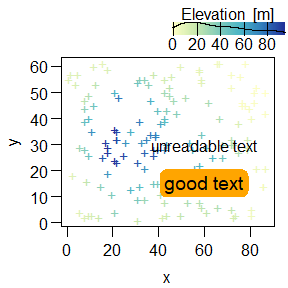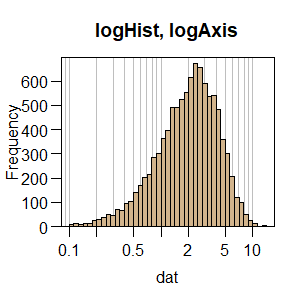Linear storage cascade (rainfall-runoff modelling): lsc, unitHydrograph, superPos, nse, rmse

# estimate parameters for Unit Hydrograph, plot data and simulation: lsc
QOBS <- dbeta(1:40/40, 3, 10) + rnorm(20,0,0.2) + c(seq(0,1,len=20), rep(1,20))
PREC <- c(1,1,3,4,5,5,4,3,1,1, rep(0,30))
lsc(PREC, QOBS, area=10, main="lsc, unitHydrograph, superPos") # , plotsim=F
##          n          k        NSE        psi
##  0.6116332 22.8333626  0.8651976  0.8651531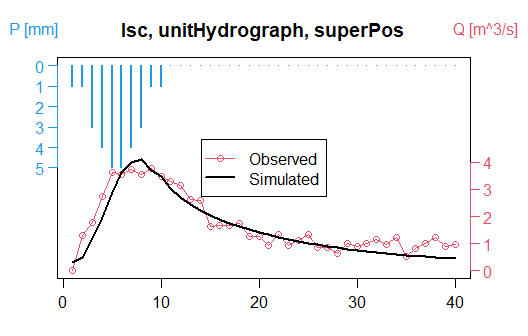Quick linear Regression: linReg
Draw circle with given radius: circle
Add transparency to existing colors: addAlpha
Fit a wide range of function types to see which one is best: mReg

a <- 1:30   ; b <- a/2.345+rnorm(30,0,3)

x <- c(1.3, 1.6, 2.1, 2.9, 4.4, 5.7, 6.6, 8.3, 8.6, 9.5)
y <- c(8.6, 7.9, 6.6, 5.6, 4.3, 3.7, 3.2, 2.5, 2.5, 2.2)
mReg(x,y, main="mReg")[,c(2,3,5:6)]
##               R2                              Formulas         a        b
## cubic       1.00 y = -0.02*x^3 + 0.42*x^2 - 3.2*x + 12 -0.020000  0.42043
## logarithmic 0.99               y = -7.3*log10(x) + 9.2 -7.302887  9.20336
## power       0.99                        y = 11*x^-0.67 10.958503 -0.67490
## square      0.98            y = 0.098*x^2 - 1.8*x + 10  0.098122 -1.75920
## exponential 0.98                   y = 9.6*e^(-0.16*x)  9.564193 -0.16080
## reciprocal  0.97                       y = 9.7/x + 1.7  9.727717  1.68322
## linear      0.91                     y = -0.73*x + 8.4 -0.725433  8.40971
## rational    0.63               y = 1/( -0.73 + 8.4*x ) -0.725433  8.40971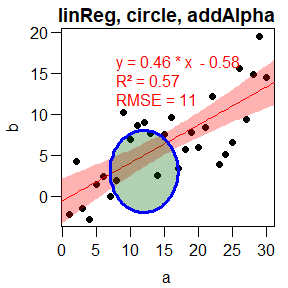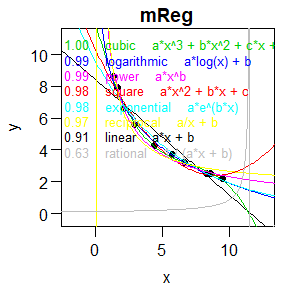Table with numbers and corresponding color: tableColVal

tableColVal(as.matrix(eurodist)[1:15,1:5], nameswidth=0.25)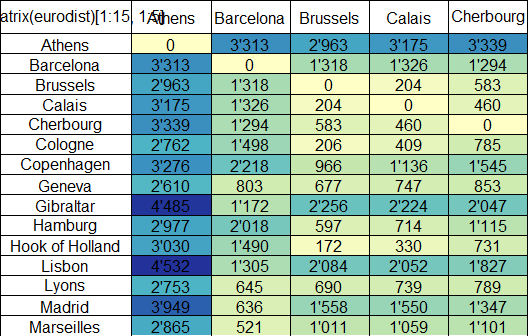Climate diagram: climateGraph

climateGraph(temp=c(-9.3,-8.2,-2.8,6.3,13.4,16.8,18.4,17,11.7,5.6,-1,-5.9),
rain=c(46,46,36,30,31,21,26,57,76,85,59,46))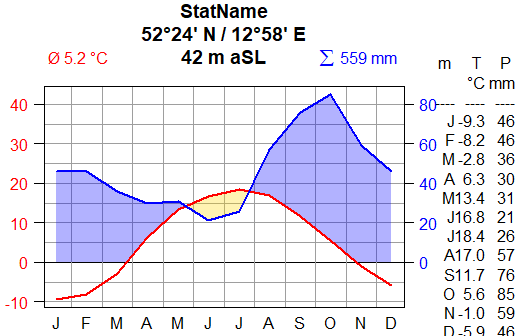TOC

## Dataframe Operations

# Convert list with vectors of unequal length to one single data.frame: l2df
eglist <- list(AB=c(6,9,2,6), CD=1:8, EF=c(-3,2) )
eglist
## $AB ##  6 9 2 6 ## ##$CD
##  1 2 3 4 5 6 7 8
##
## $EF ##  -3 2 l2df(eglist) # names are even kept ## V1 V2 V3 V4 V5 V6 V7 V8 ## AB 6 9 2 6 NA NA NA NA ## CD 1 2 3 4 5 6 7 8 ## EF -3 2 NA NA NA NA NA NA # add rows to a data.frame: addRows, insertRows MYDF <- data.frame(A=5:3, B=2:4) addRows(MYDF, 3) ## A B ## 1 5 2 ## 2 4 3 ## 3 3 4 ## 4 NA NA ## 5 NA NA ## 6 NA NA insertRows(MYDF, 2, 10:11) ## A B ## 1 5 2 ## 2 10 11 ## 3 4 3 ## 4 3 4 # Order rows in a dataframe: sortDF sortDF(USArrests[USArrests$Murder>14,], "Assault", decreasing=TRUE)
##                Murder Assault UrbanPop Rape
## Florida          15.4     335       80 31.9
## South Carolina   14.4     279       48 22.5
## Mississippi      16.1     259       44 17.1
## Louisiana        15.4     249       66 22.2
## Georgia          17.4     211       60 25.8
# truth table to test logical expressions: TFtest
TFtest(!a & !b, a&b, !(a&b))
##       a     b __ !a & !b __ a & b __ !(a & b)
## 1  TRUE  TRUE      FALSE     TRUE       FALSE
## 2  TRUE FALSE      FALSE    FALSE        TRUE
## 3  TRUE    NA      FALSE       NA          NA
## 4 FALSE  TRUE      FALSE    FALSE        TRUE
## 5 FALSE FALSE       TRUE    FALSE        TRUE
## 6 FALSE    NA         NA    FALSE        TRUE
## 7    NA  TRUE      FALSE       NA          NA
## 8    NA FALSE         NA    FALSE        TRUE
## 9    NA    NA         NA       NA          NA
# Head and tail at the same time: headtail (exception from lowerCamelCasing)
headtail(iris, n=3, na=FALSE)
##     Sepal.Length Sepal.Width Petal.Length Petal.Width   Species
## 1            5.1         3.5          1.4         0.2    setosa
## 2            4.9         3.0          1.4         0.2    setosa
## 3            4.7         3.2          1.3         0.2    setosa
## 148          6.5         3.0          5.2         2.0 virginica
## 149          6.2         3.4          5.4         2.3 virginica
## 150          5.9         3.0          5.1         1.8 virginica

TOC

## Graphics

Color palettes: seqPal, divPal, showPal
Plot simulation results as result ranges: quantileBands, ciBand

showPal(cex=3)

neff <- t(replicate(n=300, sapply(1:200, function(nn) max(rnorm(nn)))   ))
qB <- quantileBands(neff, x=1:200, smooth=7)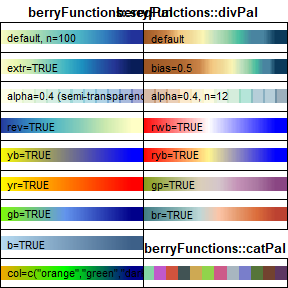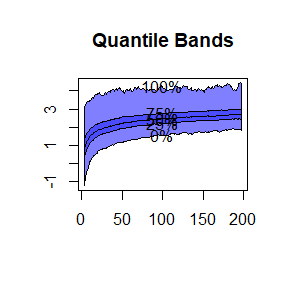Quickly plot distributions by just specifying parameters: normPlot, betaPlot

normPlot(mean=81.7, sd=11.45)
betaPlot(shape1=1.5, shape2=6)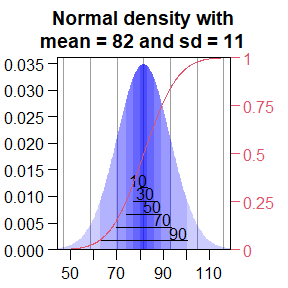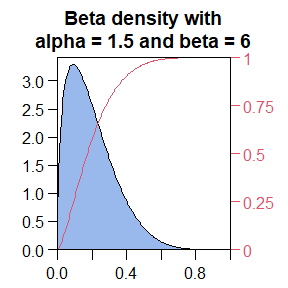Compare Beta distribution parameter effects: betaPlotComp

betaPlotComp()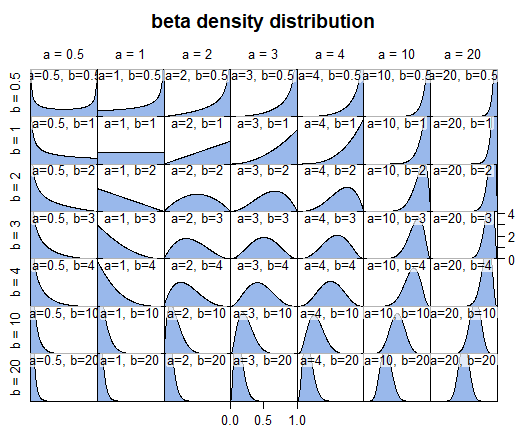Set ylim so that it does not extend below zero: lim0

val <- c(3.2, 1.8, 4.5, 8.2, 0.1, 2.9) # just some numbers
plot(val) # axes are extended by 4\% automatically, if xaxs="r"
plot(val, ylim=lim0(val), las=1) # you don't even have to set yaxs="i" ;-)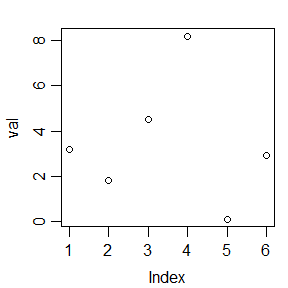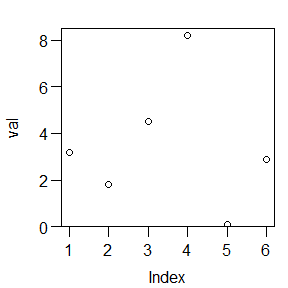Histogram with bars drawn horizontally: horizHist
Histograms for dataset split into categories: groupHist
(Uses panelDim to compute layout of panels passed to par(mfrow))

ExampleData <- rnorm(200,13,5)
hpos <- horizHist(ExampleData, col=4)
abline(h=hpos(11), col=2, lwd=2)

groupHist(chickwts, "weight", "feed", col=2, unit="gr_6")
# drop the horsebean, feed those chicks with sunflower seeds (unless you like small chicken)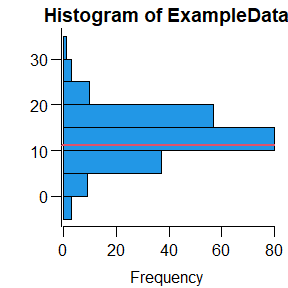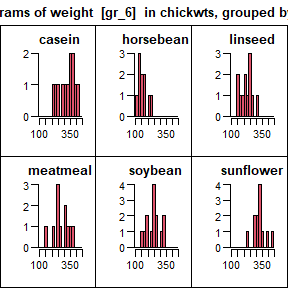A few interactive things (not shown als Graphs)
Zoom into graphics: pointZooom
Horizontal and Vertial line at point clicked on: locLine
Transformation from linear to logarithmic axis: linLogHist, linLogTrans

a <- rnorm(90); b <- rexp(90)
dev.new(record=TRUE) # turn recording on
plot(a,b, las=1)
pointZoom(a,b) # scroll through the plots (Pg Up and Pg Dn) to unzoom again.

locLine()

x <- rlnorm(700, m=3)
dev.new(record=TRUE) # scroll through the plots (Pg Up and Pg Dn)...
linLogHist(x, xlab="ddd", breaks=30, yaxt="n", freq=FALSE)

Moving average with overlapping windows: movAv, movAvLines
Funnel plot for proportional Data: funnelPlot

plot(a, type="l", pch=16, las=1)
lines(movAv(a), col=2, lwd=3)
movAvLines(y=a, lwd=3)

X <- c(2, 224,  54,  505, 1,  5, 236,  92,  3, 0) # successful events
N <- c(2, 400, 100, 1000, 2, 10, 500, 200, 10, 2) # possible succeses
funnelPlot(X,N, letters[1:10])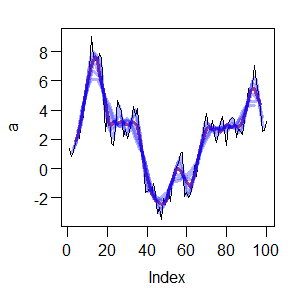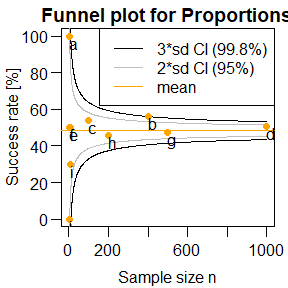Get nice values and labels to write at logarithmic axes: logVals, logAxis
Label time axis in date-regular intervals: monthLabs, monthAxis

exdat <- 10^runif(50, -1, 2)
plot(exdat, log="y", yaxt="n")
logAxis(side=2) # invisibly returns values and labels
points(exdat, pch=16)

plot(as.Date("2013-04-25")+0:500, cumsum(rnorm(501)), type="l", xaxt="n", ann=FALSE)
dummy <- monthAxis(side=1)
str(dummy)
## List of 4
##  $mlabs: Date[1:18], format: "2013-04-15" "2013-05-15" ... ##$ ylabs: Date[1:2], format: "2013-08-18" "2014-05-15"
##  $mtics: Date[1:19], format: "2013-04-01" "2013-05-01" ... ##$ ytics: Date[1:1], format: "2014-01-01"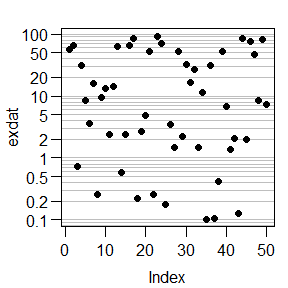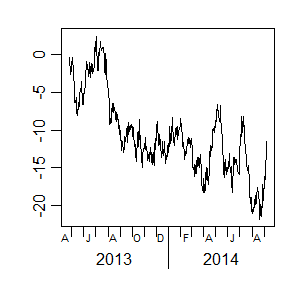TOC

## Hydrology

Extreme value Statistics (e.g. for flood risk estimation): moved to https://github.com/brry/extremeStat

# superposition of precipitation to simulate Q from P: superPos
N <- c(9,5,2,14,1,3) # [mm/hour]
UH <- c(0.1, 0.4, 0.3, 0.1, 0.1) # [1/h]
superPos(N, UH)
##   0.9 4.1 4.9 4.6 7.7 5.6 3.1 2.4 0.4 0.3
# calculate continuous UH with given n and k: unitHydrograph
plot(0:40, unitHydrograph(n=2,  k=3, t=0:40), type="l")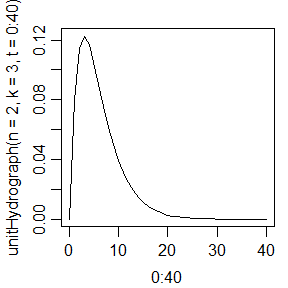# Nash-Sutcliffe and kling-gupta efficiency: nse + kge
QSIM <- lsc(PREC, QOBS, area=10, returnsim=TRUE, plot=FALSE)
## Warning in lsc(PREC, QOBS, area = 10, returnsim = TRUE, plot = FALSE): sum of UH
## is not 1, probably the time should be longer
nse(QOBS, QSIM)
##  0.8652
kge(QOBS, QSIM)
##  0.88685
# Root Mean Squared Error, e.g. to be minimized: rmse
rmse(QOBS, QSIM)
##  0.38461
# R squared (coefficient of determination): rsquare
rsquare(QOBS, QSIM)
##  0.89574

TOC

## Programming

tmessage, twarning, tstop: explicit tracing of messages, warnings and errors:

lower <- function(a, s) {tmessage("some stuff with ", a+10, skip=s); a}
upper <- function(b, skip=0) lower(b+5, skip)
upper(3) 
## tools::buildVignettes ->  upper -> lower: some stuff with 18
##  8

tryStack: tracing any message / warning error in other people’s code, can also log to a file:

lower <- function(a) {message("fake message, a = ", a); a+10}
middle <- function(b) {plot(b, main=b) ; warning("fake warning, b = ", b); lower(b) }
upper <- function(c) {cat("printing c:", c, "\n") ; middle(c)}
tryStack(upper("42") )
## printing c: 42
## Message: fake message, a = 42
## -- tryStack sys.calls: tools::buildVignettes -> engine$weave -> vweave_rmarkdown -> rmarkdown::render -> knitr::knit -> process_file -> withCallingHandlers -> process_group -> process_group.block -> call_block -> block_exec -> eng_r -> in_input_dir -> in_dir -> evaluate -> evaluate::evaluate -> evaluate_call -> timing_fn -> handle -> withCallingHandlers -> withVisible -> eval_with_user_handlers -> eval -> eval -> tryStack -> upper -> middle -> lower -> message -> message("fake message, a = ", a)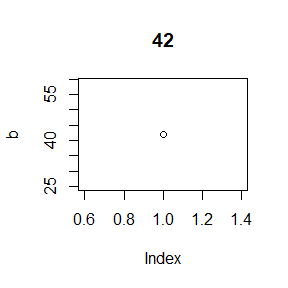## tryStack error in a + 10: non-numeric argument to binary operator ## -- tryStack sys.calls: tools::buildVignettes -> engine$weave -> vweave_rmarkdown -> rmarkdown::render -> knitr::knit -> process_file -> withCallingHandlers -> process_group -> process_group.block -> call_block -> block_exec -> eng_r -> in_input_dir -> in_dir -> evaluate -> evaluate::evaluate -> evaluate_call -> timing_fn -> handle -> withCallingHandlers -> withVisible -> eval_with_user_handlers -> eval -> eval -> tryStack -> upper -> middle -> lower -> a + 10

TOC

## Miscellaneous

# distance between two points on a plane: distance
A <- c(3,  9,-1)  ;  B <- c(7, -2, 4)
plot(A,B); points(3,5, col=2, pch=16); segments(3,5, A,B)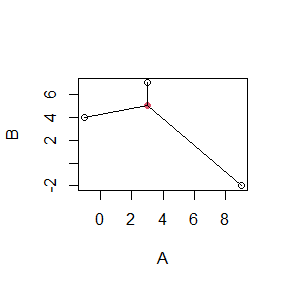distance(A,B, 3,5)
##  2.0000 9.2195 4.1231
# remove leading and trailing white space: removeSpace
s <- c("space at end     ", "  white at begin", "  both ", " special ^  ")
removeSpace(s)
## Warning in removeSpace(s): since R 3.2.0 (April 2015), there is trimws().
## removeSpace() will be removed from berryFunctions one day.
##  "space at end"   "white at begin" "both"           "special ^"
# sequence given by range or vector of values: seqR
seqR(range=c(12,6), by=-2)
##  12 10  8  6
seqR(rnorm(20), len=7)
##  -1.90100 -1.31629 -0.73159 -0.14688  0.43782  1.02253  1.60723
# Rescale values to another range: rescale
rescale(10:15, from=200, to=135)
##  200 187 174 161 148 135
# Show memory size of the biggest objects in MB: lsMem
lsMem(n=5)
##      neff       dat        qB      hpos    middle sum rest:
##  0.480216  0.079832  0.020992  0.007472  0.007024  0.022184
# extract pdf link from google search result url: googleLink2pdf
"%2Fdoc%2Fmanuals%2FR-intro.pdf&ei=Nyl4UfHeOIXCswa6pIC4CA",
"&usg=AFQjCNGejDwPlor4togQZmQEQv72cK9z8A&bvm=bv.45580626,d.Yms")
googleLink2pdf(Link)
##  "http://cran.r-project.org/doc/manuals/R-intro.pdf"
# Create a number of 999 strings with spaces for reading files: na9
na9()[c(1:4,13,30)]
##  "-9999"     "-999"      "-9,99"     "-9,999"    "-999,0000" " -999"

A few things not executed for this document:

# Separate lists with arguments for functions: owa
?owa # the example section has a good - wait for it - example!

# install.package and require in one single function: require2

# Write a file with a Roxygen-compatible function structure,
# making it easy to add new functions to the package: createFun
createFun(myNewFunction, package="extremeStat", path="S:/Dropbox")

# Open the source code of a function on github: funSource
funSource("smoothLines")

# Install a package from github without dependencies: instGit
instGit("brry/shapeInteractive")

# concatenate textfiles contents unchanged into one file: combineFiles
cat("This is Sparta.\nKicking your face.", file="BujakashaBerry1.txt")
cat("Chuck Norris will roundhousekick you.", file="BujakashaBerry2.txt")
combineFiles(inFiles=paste0("BujakashaBerry", 1:2, ".txt"),
outFile="BujakashaBerry3.txt")

# wish neRds a happy new year: yearSample
yearSample(2016)
# Have a nerdy
set.seed(12353); sample(0:9,4,T)

# generate name from "random" sample: nameSample
nameSample("berry")
# Kind regards from
set.seed(8833277); paste(sample(letters,5,rep=T),collapse='')
##  "berry"

TOC

Explore the other possibilities of the package by reading the function help files.
Any Feedback on this package (or this vignette) is very welcome via github or !